xSpanning tree (mathematics)Encyclopedia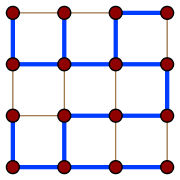In the mathematical
Mathematics
Mathematics is the study of quantity, space, structure, and change. Mathematicians seek out patterns and formulate new conjectures. Mathematicians resolve the truth or falsity of conjectures by mathematical proofs, which are arguments sufficient to convince other mathematicians of their validity...

field of graph theory
Graph theory
In mathematics and computer science, graph theory is the study of graphs, mathematical structures used to model pairwise relations between objects from a certain collection. A "graph" in this context refers to a collection of vertices or 'nodes' and a collection of edges that connect pairs of...

, a spanning tree T of a connected, undirected graph G is a tree
Tree (graph theory)
In mathematics, more specifically graph theory, a tree is an undirected graph in which any two vertices are connected by exactly one simple path. In other words, any connected graph without cycles is a tree...

composed of all the vertices and some (or perhaps all) of the edges of G. Informally, a spanning tree of G is a selection of edges of G that form a tree spanning every vertex. That is, every vertex
Vertex (graph theory)
In graph theory, a vertex or node is the fundamental unit out of which graphs are formed: an undirected graph consists of a set of vertices and a set of edges , while a directed graph consists of a set of vertices and a set of arcs...

lies in the tree, but no cycles
Cycle (graph theory)
In graph theory, the term cycle may refer to a closed path. If repeated vertices are allowed, it is more often called a closed walk. If the path is a simple path, with no repeated vertices or edges other than the starting and ending vertices, it may also be called a simple cycle, circuit, circle,...

(or loops) are formed.
On the other
hand, every bridge
Bridge (graph theory)
In graph theory, a bridge is an edge whose deletion increases the number of connected components. Equivalently, an edge is a bridge if and only if it is not contained in any cycle....

of G must belong to T.

A spanning tree of a connected graph G can also be defined as a maximal set of edges of G that contains no cycle, or as a minimal set of edges that connect all vertices.

In certain fields of graph theory it is often useful to find a minimum spanning tree
Minimum spanning tree
Given a connected, undirected graph, a spanning tree of that graph is a subgraph that is a tree and connects all the vertices together. A single graph can have many different spanning trees...

of a weighted graph. Other optimization problems on spanning trees have also been studied, including the maximum spanning tree, the minimum tree that spans at least k vertices, the minimum spanning tree with at most k edges per vertex (Degree-Constrained Spanning Tree), the spanning tree with the largest number of leaves (closely related to the smallest connected dominating set
Dominating set
In graph theory, a dominating set for a graph G =  is a subset D of V such that every vertex not in D is joined to at least one member of D by some edge...

), the spanning tree with the fewest leaves (closely related to the Hamiltonian path problem
Hamiltonian path problem
In the mathematical field of graph theory the Hamiltonian path problem and the Hamiltonian cycle problem are problems of determining whether a Hamiltonian path or a Hamiltonian cycle exists in a given graph . Both problems are NP-complete...

), the minimum diameter spanning tree, and the minimum dilation spanning tree.

## Fundamental cycles

Adding just one edge to a spanning tree will create a cycle; such a cycle is called a fundamental cycle. There is a distinct fundamental cycle for each edge; thus, there is a one-to-one correspondence between fundamental cycles and edges not in the spanning tree. For a connected graph with V vertices, any spanning tree will have V-1 edges, and thus, a graph of E edges will have E-V+1 fundamental cycles. For any given spanning tree these cycles form a basis for the cycle space
Cycle space
In graph theory, an area of mathematics, a cycle space is a vector space defined from an undirected graph; elements of the cycle space represent formal combinations of cycles in the graph....

.

Dual to the notion of a fundamental cycle is the notion of a fundamental cutset. By deleting just one edge of the spanning tree, the vertices are partitioned into two disjoint sets. The fundamental cutset is defined as the set of edges that must be removed from the graph G to accomplish the same partition. Thus, there are precisely V-1 fundamental cutsets for the graph, one for each edge of the spanning tree.

The duality between fundamental cutsets and fundamental cycles is established by noting that cycle edges not in the spanning tree can only appear in the cutsets of the other edges in the cycle; and vice versa: edges in a cutset can only appear in those cycles containing the edge corresponding to the cutset.

## Spanning forests

A spanning forest is a type of subgraph that generalises the concept of a spanning tree. However, there are two definitions in common use. One is that a spanning forest is a subgraph that consists of a spanning tree in each connected component
Connected component (graph theory)
In graph theory, a connected component of an undirected graph is a subgraph in which any two vertices are connected to each other by paths, and which is connected to no additional vertices. For example, the graph shown in the illustration on the right has three connected components...

of a graph. (Equivalently, it is a maximal cycle-free subgraph.) This definition is common in computer science and optimization. It is also the definition used when discussing minimum spanning forests, the generalization to disconnected graphs of minimum spanning trees. Another definition, common in graph theory
Graph theory
In mathematics and computer science, graph theory is the study of graphs, mathematical structures used to model pairwise relations between objects from a certain collection. A "graph" in this context refers to a collection of vertices or 'nodes' and a collection of edges that connect pairs of...

, is that a spanning forest is any subgraph that is both a forest (contains no cycles) and spanning (includes every vertex).

## Counting spanning trees

The number t(G) of spanning trees of a connected graph is an important
invariant
Invariant (mathematics)
In mathematics, an invariant is a property of a class of mathematical objects that remains unchanged when transformations of a certain type are applied to the objects. The particular class of objects and type of transformations are usually indicated by the context in which the term is used...

. In some cases, it is easy to calculate t(G) directly. It is also widely used in data structures in different computer languages.
For example, if G is itself a tree, then t(G)=1, while if G is the
cycle graph
Cycle graph
In graph theory, a cycle graph or circular graph is a graph that consists of a single cycle, or in other words, some number of vertices connected in a closed chain. The cycle graph with n vertices is called Cn...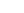with n vertices, then t(G)=n.
For any graph G, the number t(G) can be calculated
using Kirchhoff's matrix-tree theorem
Kirchhoff's theorem
In the mathematical field of graph theory Kirchhoff's theorem or Kirchhoff's matrix tree theorem named after Gustav Kirchhoff is a theorem about the number of spanning trees in a graph...

(follow the link for an explicit example using the theorem).

Cayley's formula is a formula for the number of spanning trees in the complete graph
Complete graph
In the mathematical field of graph theory, a complete graph is a simple undirected graph in which every pair of distinct vertices is connected by a unique edge.-Properties:...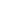with n vertices. The formula states that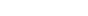. Another way of stating Cayley's formula is that there are exactly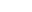labelled trees with n vertices. Cayley's formula can be proved using Kirchhoff's matrix-tree theorem or via the
Prüfer code.

If G is the complete bipartite graph
Complete bipartite graph
In the mathematical field of graph theory, a complete bipartite graph or biclique is a special kind of bipartite graph where every vertex of the first set is connected to every vertex of the second set.- Definition :...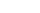, then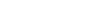, while if G is the n-dimensional hypercube graph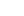, then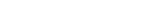.
These formulae are also consequences of the matrix-tree theorem.

If G is a multigraph
Multigraph
In mathematics, a multigraph or pseudograph is a graph which is permitted to have multiple edges, , that is, edges that have the same end nodes. Thus two vertices may be connected by more than one edge....

and e is an edge of G, then the number t(G) of spanning trees of G satisfies the deletion-contraction recurrence
t(G)=t(G-e)+t(G/e), where G-e is the multigraph obtained by deleting e
and G/e is the contraction
Edge contraction
In graph theory, an edge contraction is an operation which removes an edge from a graph while simultaneously merging together the two vertices it previously connected. Edge contraction is a fundamental operation in the theory of graph minors...

of G by e, where multiple edges arising from this contraction are not deleted.

## Uniform spanning trees

A spanning tree chosen randomly from among all the spanning trees with equal probability is called a uniform spanning tree (UST). This model has been extensively researched in probability
Probability
Probability is ordinarily used to describe an attitude of mind towards some proposition of whose truth we arenot certain. The proposition of interest is usually of the form "Will a specific event occur?" The attitude of mind is of the form "How certain are we that the event will occur?" The...

and mathematical physics
Mathematical physics
Mathematical physics refers to development of mathematical methods for application to problems in physics. The Journal of Mathematical Physics defines this area as: "the application of mathematics to problems in physics and the development of mathematical methods suitable for such applications and...

.

## Algorithms

The classic spanning tree algorithm, depth-first search
Depth-first search
Depth-first search is an algorithm for traversing or searching a tree, tree structure, or graph. One starts at the root and explores as far as possible along each branch before backtracking....

(DFS), is due to Robert Tarjan
Robert Tarjan
Robert Endre Tarjan is a renowned American computer scientist. He is the discoverer of several important graph algorithms, including Tarjan's off-line least common ancestors algorithm, and co-inventor of both splay trees and Fibonacci heaps. Tarjan is currently the James S...

. Another important algorithm is based on breadth-first search
In graph theory, breadth-first search is a graph search algorithm that begins at the root node and explores all the neighboring nodes...

(BFS).

Parallel algorithms typically take different approaches than BFS or DFS. Halperin and Zwick designed an optimal randomized parallel algorithm that runs in O(log n) time with high probability on EREW PRAM.http://citeseer.ist.psu.edu/652374.html The Shiloach-Vishkin algorithm, due to Yossi Shiloach and Uzi Vishkin
Uzi Vishkin
Uzi Vishkin is a computer scientist at the University of Maryland, College Park, where he is Professor of Electrical and Computer Engineering at the University of Maryland Institute for Advanced Computer Studies . Uzi Vishkin is known for his work in the field of parallel computing...

, is the basis for many parallel implementations.http://citeseer.ist.psu.edu/context/74288/0 Bader and Cong's algorithm is shown to run fast in practice on a variety of graphs.http://portal.acm.org/citation.cfm?id=1196220

The most common distributed algorithm is the Spanning Tree Protocol
Spanning tree protocol
The Spanning Tree Protocol is a network protocol that ensures a loop-free topology for any bridged Ethernet local area network. The basic function of STP is to prevent bridge loops and ensuing broadcast radiation...

, used by OSI link layer